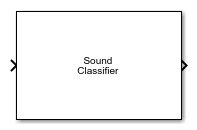# Sound Classifier

Classify sounds in audio signal

Since R2021b

•Libraries:
Audio Toolbox / Deep Learning

## Description

The Sound Classifier block uses YAMNet to classify audio segments into sound classes described by the AudioSet ontology. The Sound Classifier block combines necessary audio preprocessing and YAMNet network inference. The block returns predicted sound labels, predicted scores from the sounds, and class labels for predicted scores.

## Ports

### Input

expand all

Sound data to classify, specified as a one-channel signal (column vector). If Sample rate of input signal (Hz) is 16e3, there are no restrictions on the input frame length. If Sample rate of input signal (Hz) is different from 16e3, then the input frame length must be a multiple of the decimation factor of the resampling operation that the block performs. If the input frame length does not satisfy this condition, the block throws an error message with information on the decimation factor.

Data Types: `single` | `double`

### Output

expand all

Predicted sound label, returned as an enumerated scalar.

Data Types: `enumerated`

Predicted activation or score values for each supported sound label, returned as a 1-by-521 vector, where 521 is the number of classes in YAMNet.

Data Types: `single`

Class labels for predicted scores, returned as a 1-by-521 vector.

Data Types: `enumerated`

## Parameters

expand all

Specify the sample rate of the input signal as a positive scalar in Hz. If the sample rate is different from 16e3, then the block resamples the signal to 16e3, which is the sample rate that YAMNet supports.

Data Types: `single` | `double`

Specify the overlap percentage between consecutive mel spectrograms as a scalar in the range [0 100).

Data Types: `single` | `double`

Enable the output port sound, which outputs the classified sound.

Enable the output ports scores and labels, which output all predicted scores and associated class labels.

## Block Characteristics

 Data Types `double` | `single` Direct Feedthrough `no` Multidimensional Signals `no` Variable-Size Signals `no` Zero-Crossing Detection `no`

## Algorithms

expand all

The Sound Classifier block algorithm consists of two steps:

1. Preprocessing –– YAMNet specific preprocessing. Generates mel spectrograms.

2. Prediction –– Predicting the sounds, scores, and labels of the input signal using the YAMNet sound classification network.

## Version History

Introduced in R2021b